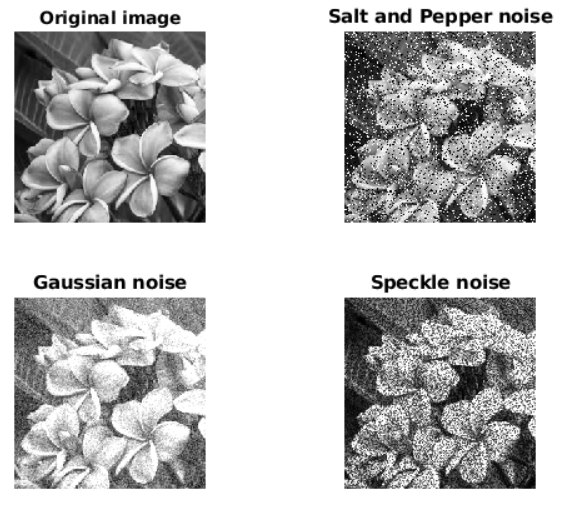# Noise addition using in-built Matlab function

Noise in an image: Digital images are prone to various types of noise that makes the quality of the images worst. Image noise is random variation of brightness or color information in the captured image. Noise is basically the degradation in image signal caused by external sources such as camera. Images containing multiplicative noise have the characteristic that the brighter the area the noisier it. But mostly it is additive. There are several ways to introduce noise into an image, depending on how the image is created. For example:

• If the image is scanned from a photograph made on film, the film grain can be the source of noise. Noise can also be the result of damage to the film, or be introduced by the scanner fluctuations itself.
• If the image is acquired directly in digital format, the mechanism for gathering the data (such as a CCD detector) can introduce noise.
• Electronic transmission of digital image data can also introduce noise.

To simulate the effects of some of the problems listed above, the toolbox provides the in-built imnoise function in MATLAB, which you can use to add various types of noise to an image.

Various kind of noise that can be introduced by MATLAB inbuilt function are:

• Salt and Pepper noise: Salt and pepper noise refers to a wide variety of processes that result in the image degradation. In Salt and Pepper noise only few pixels are noisy, but they are very noisy. The effect is similar to sprinkling white and black dots on the image.
`X = imnoise(Image, 'salt & pepper', percentage_distortion)`

Above in-built function adds “salt and pepper” noise to an image named Image, where percentage_distortion is the noise density. The default for percentage_distortion is 0.05.

• Gaussian noise: Gaussian Noise is a statistical noise having a probability density function equal to normal distribution, also known as Gaussian Distribution. It is also called electronic noise because it arises in amplifiers or detectors.
`X = imnoise(Image, 'gaussian', percentage_distortion)`
• Speckle noise: Speckle is a noise that inherently exists in an image and degrades its quality. Speckle noise can be generated by multiplying random pixel values with different pixels of an image.
`X = imnoise(Image, 'speckle', percentage_distortion)`

Below is the Matlab code for adding noise in an image:

 `% For adding various kind of noise ` `clear; ` `I = rgb2gray(imread(``"flowers.jpg"``)); ` `subplot(2, 2, 1), ` `imshow(I); ` `title(``"Original image"``); ` ` `  `% adding salt and pepper noise ` `s = imnoise(I, ``"salt & pepper"``, 0.20); ` `subplot(2, 2, 2), ` `imshow(s); ` `title(``"Salt and Pepper noise"``); ` ` `  `% adding Gaussian noise ` `g = imnoise(I, ``"gaussian"``, 0.20); ` `subplot(2, 2, 3), ` `imshow(g); ` `title(``"Gaussian noise"``); ` ` `  `% adding Speckle noise ` `sp = imnoise(I, ``"speckle"``, 0.20); ` `subplot(2, 2, 4), ` `imshow(sp); ` `title(``"Speckle noise"``); `

Output :Whether you're preparing for your first job interview or aiming to upskill in this ever-evolving tech landscape, GeeksforGeeks Courses are your key to success. We provide top-quality content at affordable prices, all geared towards accelerating your growth in a time-bound manner. Join the millions we've already empowered, and we're here to do the same for you. Don't miss out - check it out now!